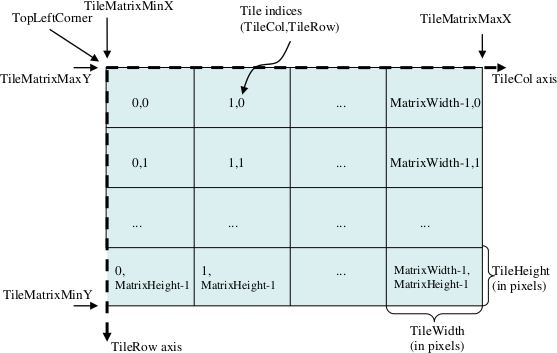# WMTS: Few Things I Want To Remember

• Used to serve prepared rectangular tiles; this means you are limited by web server speed rather than map server speed
• Several ways to retrieve tiles are defined: KVP and REST are mandatory, SOAP is optional
• Does not allow layer combination; additional tile matrix would have to be created
• GetCapabilities, GetTile and GetFeatureInfo requests are defined
• tile
• rectangular representation of space
• defined by tile and row indices
• tile matrix
• set of tiles for a given scale
• defined with:
• tile size derived from standardized pixel size (0.28 × 0.28 mm)
• tile width and tile height (px)
• left upper corner coordinates
• matrix width and height as number of tiles
• tile matrix set
• set of tile matrices for different scales

### Total count of tile matrices

`nTileMatrices × nTiledStyles × nTiledFormats (if no dimensions are defined)`

### Total count of tiles in a tile matrix

`matrixWidth × matrixHeight`

### Other equations

• `pixelSpan = scaleDenominator × 0.28 103 / metersPerUnit(crs);`
• `tileSpanX = tileWidth × pixelSpan;`
• `tileSpanY = tileHeight × pixelSpan;`
• `tileMatrixMaxX = tileMatrixMinX + tileSpanX × matrixWidth;`
• `tileMatrixMinY = tileMatrixMaxY - tileSpanY × matrixHeight;`